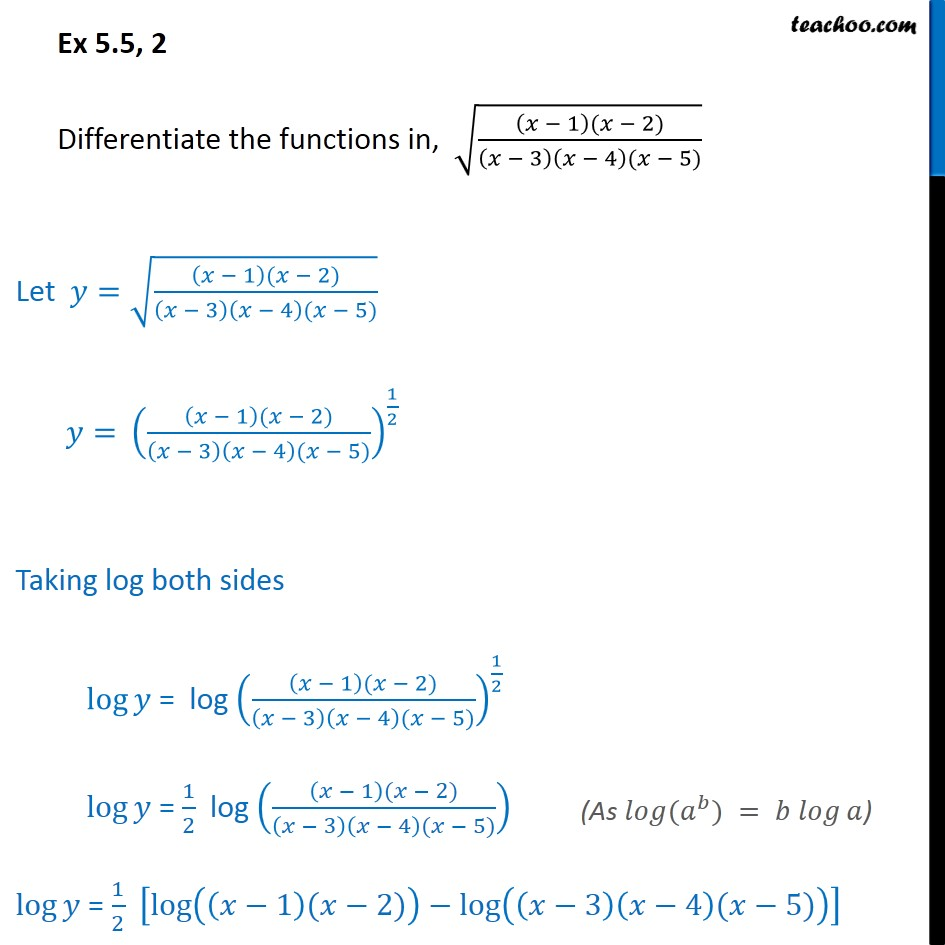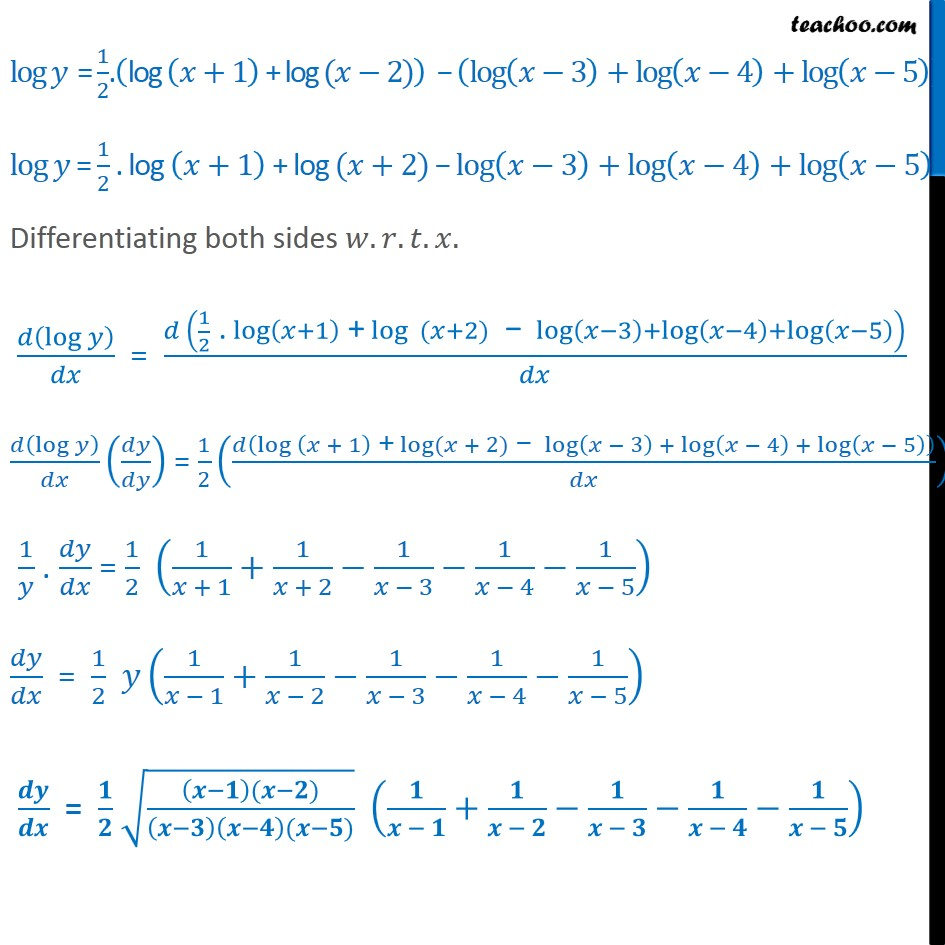1. Chapter 5 Class 12 Continuity and Differentiability
2. Serial order wise
3. Ex 5.5

Transcript

Ex 5.5, 2 Differentiate the functions in, 1 ( 2) 3 4 ( 5) Let = 1 ( 2) 3 4 ( 5) = 1 ( 2) 3 4 ( 5) 1 2 Taking log both sides log = log 1 ( 2) 3 4 ( 5) 1 2 log = 1 2 log 1 ( 2) 3 4 ( 5) log = 1 2 log 1 ( 2) log 3 4 ( 5) log = 1 2 . log +1 + log ( 2) log 3 + log 4 + log 5 log = 1 2 . log +1 + log ( +2) log 3 + log 4 + log 5 Differentiating both sides . . . . log = 1 2 . log +1 + log ( +2) log 3 + log 4 + log 5 log = 1 2 log + 1 + log ( + 2) log 3 + log 4 + log 5 1 . = 1 2 1 + 1 + 1 + 2 1 3 1 4 1 5 = 1 2 1 1 + 1 2 1 3 1 4 1 5 = ( ) ( ) +

Ex 5.5